# Puzzles with 4 numbers, and the amazing power of powers

A fun puzzle is to choose a number between 1 and 10, then using that number exactly four times in an expression involving basic mathematical operations, i.e. at leastbut also possibly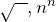, see what values you can create.

For instance, can you get all the numbers from 1 to 10 using just four 2s? Have a try using pen and paper, or the activities at Four Twos, or Four Numbers. This is an excellent way to practice mathematical thinking, order of operations, problem solving. Think about which numbers were easier to use (as either starting values or target values) and which were harder. What things do you think make some easy and some hard?

## Four Twos

The Four Twos version is my favourite.

• Can you get every number from 1 to 10? Don’t try too hard for 7… it’s not possible.
• What is the largest value you can construct?
• Did you realise the game lets you put two 2s together to make 22? What further values can you construct using this feature?

Let’s explore making large values. It is clear that raising to a power is the most effective way to make a large number, and using just the four 2s, the largest value is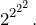To calculate how big this “tower of powers” actually is, you start from the right and work left:But remember, we can join the 2s together to get 22, 222 etc. Might this let us make even larger numbers?

If you’ve given the four 2s puzzle a good go, you will have found that the way to get really large numbers is to get a really large power. The largest possible power will be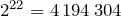, and so the largest number is. But just how big is this? Well, we can get some idea by thinking about the power. Every three times we multiply by 2, the value increases by a factor of 8, and that is not too different from 10, so we can estimate that every three 2s we multiply approximately adds an extra digit to the answer. Therefore, we would expect something similar, but a bit less than,million digits. The exact value has 1 262 612 digits — you can see them all at The Mathenæum. That’s an incredibly big number to make from just four 2s, and illustrates the amazing power of powers! It’s worth a look.

## Bamboozler: Only 1.2 million digits? You ain’t seen nothing yet!

Obviously with four 3s we can get even larger. Are you wondering just how much larger? We can reason as before —is approximately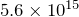, and eachgives close to one extra digit in the answer, so we’d expect a little less than half this number of digits, say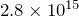digits. The exact value is 2 652 345 952 577 568 digits. Wow! That’s incredible. Just by going from 2s to 3s, we’ve gone from 1.2 million digits to more than 2½ thousand million million digits!

I think we need to pause here and take a deep breath. 2½ thousand million million (a thousand million million is usually called a quadrillion) is a big number. A very big number — more than 300 000 times the world population. But this is not the value of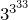, it is the number of digits in. One million has only 6 digits, a billion only 9, a trillion only 12. Our number has quadrillions of digits! It is estimated that the total number of atoms in the universe is roughly 1080 — a measly 80 digit number. A number with over a million digits, such asis incredibly large, but can still be easily printed out. If we were to printone hundred thousand digits to a page and share the pages among the Earth’s population, every man, woman and child on Earth would need to hold 4 pages.

But we have only moved from 2s to 3s. We have all the way up to 9 to consider. Before we do though, let’s take a slight detour.

About 80 years ago, the nine-year-old nephew of a mathematician was asked to think of a name for the number written as a 1 followed by 100 zeroes. He came up with googol, which was subsequently published and is now in common use. The related number, 1 followed by a googol zeroes, he called a googolplex. This number is unimaginably huge. Even if our digits were microscopically small and we filled the entire universe with them, we would get nowhere near writing out a googolplex. And even if we produced billions of digits a second, for billions of times the age of the universe, we would barely get started printing it out! We would need to write maybe 10 digits every second on every atom in the universe for the entire lifetime of the universe to get close to writing out a googolplex.

If that’s not bamboozling, I don’t know what is.

Surely we cannot get anywhere close to this number with just four digits? Well, let’s see. To get the largest value possible with four 2s, we used 22 rather than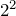in our powers because. Similarly. But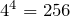is bigger than 44, and so that trick is no longer helpful. The biggest number we can get with four 4s is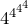and this number absolutely dwarfs a googolplex! It is approximately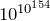while a googolplex is a paltry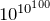. We’ve just discussed how completely and utterly impossible it is to write out a googolplex, but to write out this number would be like writing a googolplex one million trillion trillion trillion trillion times. And that’s just with four 4s. Can you believe how this thing is growing?

Skipping ahead to four 9s, the number of digits in our power tower of four 9s is approximately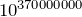. That means just to count the number of digits in our answer needs a number that itself has 370 million digits. Three. Hundred. And. Seventy. Million. Digits. Just for the number of digits! The number itself is something like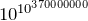, but what does that even mean???

Don’t ask me — I am so completely bamboozled that I fear my brain will shut down. I’m heading off now to have a nice lie down…Mr Wessen

Sometime teacher, sometime programmer, full time mathematician.